## 1. Energy of a particle.

Physicists in 18th and 19th centuries, developing the newtonian Mechanics, defined the mechanical energy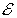of a particle (a point body with inertial mass m).

In particular, they stated that, if a particle is at the point P of a conservative field, a scalar function of the position r of P can be defined, usually denoted by V(r), said potential, and the mechanical energyof the particle in position r and with velocity v is given by the sum of two functions:

• the kinetic energy K, which depends on inertial mass m and on the velocity v;
• the potential energy U, which depends on the potential V(r) and on a property Q, which can be said generally the charge of the particle and which expresses the grade of its sensibility to the field;
Q may be, with respect to the field, the gravitational charge i.e. the gravitational mass or the electric charge or other kind of charge.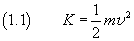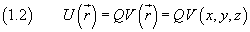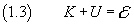If we introduce the quantity p, said momentum of the particle, defined as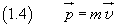the (1.1) may be written as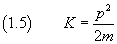and the (1.3) may be written as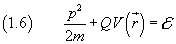From Newton's laws it follows that the energyof a particle moving in a conservative field is constant.

Because the energy is condensed in the particle, the motion of the particle implies a flow of energy.

For example, the energy of a bullet at the mouth of a gun flows due to the motion of the bullet and when the bullet hits the target, if the collision is anelastic, the energy spreads within the target as heat.

Therefore we can say that the energy can propagate due the motion of one or several particles.